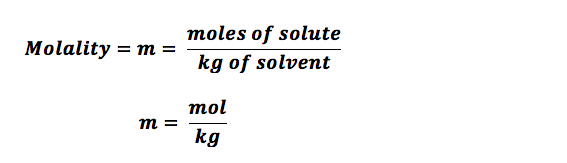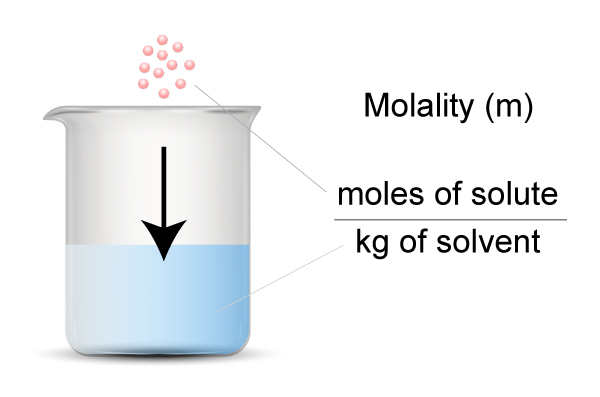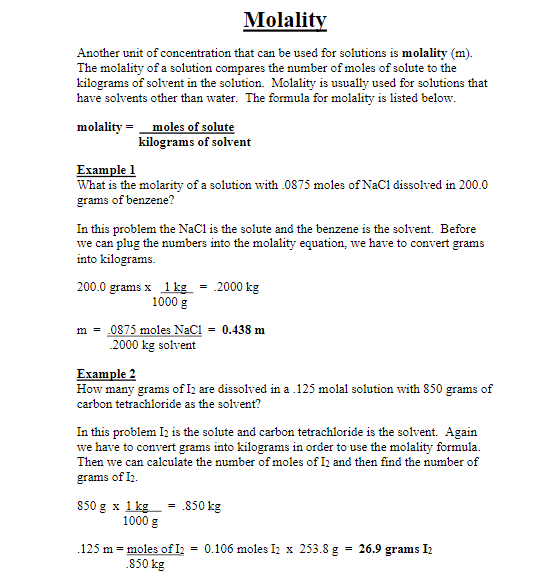# Molality Study Guide

INTRODUCTION

What does one mean by molality? What is molality in chemistry? Molality is a characteristic of any solution, defined as the total number of moles of solute present per kilogram of solvent.

A solution primarily consists of two components- solvent and solute. There are various ways and calculations to express the concentration of solutions, like molarity, normality, molality, volume and weight percentage, and formality alongside part per million.

### WHAT IS MOLARITY?

Molar concentration measures the chemical species concentration, particularly a solute in a solvent, in terms of the amount of substance per unit volume of solution.

``                               M = n/v ``

where M equals molar concentration, N is moles of solute, and v is liters of solution.

### MOLALITY AND its FORMULA

The molality of a solution is often defined by ‘m’ and is the amount of solute present in moles, divided by the total mass in a kilogram of the solvent.Compared to other molar concentrations or even mass concentrations, the solution preparation of a given molality is easier. Besides, in some weak aqueous solutions, the value of molality and molarity are almost similar. This is because 1 kg of water takes up 1 liter of volume at normal temperature, and the negligible amount of solute has significantly less effect on the overall volume.

Unlike molarity which completely depends on solution volume, molality is a intensive property and is only calculated as moles of a solute divided by the total kilograms of the solvent. Molarity varies greatly because of variation in volume due to external factors like temperature and pressure. When working with a wide temperature range, molality is taken as the standard calculation.

For example, we know that salt easily gets dissolved in water. Does that mean you cannot calculate the molality? If you only know the basics, including the mass of salt and water, the molality could be easily determined.### Difference between Molarity and Molality

Molarity Molality
The total number of moles of solute in a solution per liter is known as its molarity. The quantity of moles of a solute per kilogram of solvent is known as a solution’s molality
Molarity is measured in units of M or mol/L. Molality is measured in m or mol/k
M represents it. m represents it.
It is based on the solvent’s volume, temperature, and pressure. It is independent of the solvent’s volume, temperature, and pressure.
It is independent of the solvent’s mass. It is based on the solvent’s mass

### Molality Examples

For example, when table salt is dissolved in water, water serves as both the solution and the solute. Sodium chloride weights 58.44 grams per mole. One molar solution, or 1M, is created by dissolving 58.44 grams of sodium chloride in one litre of water.

• One advantage of using molality as a concentration indicator is that, as was already noted, molality exclusively depends on the mass of the solute and the solvent. It means that, unlike in the case of solutions made volumetrically, these parameters are not impacted by variations in temperature or even pressure.
• The molality of one solute in a solution is not reliant on the presence of other solutes, which makes it very helpful. The lack of applicability in situations when there is no pure material in a combination is one of its disadvantages.
• For instance, alloys or mixes like alcohol and water. Any material might serve as the solvent in this case.

### Molarity

The total number of moles of solute in a particular solution’s molarity is expressed as moles of solute per liter of solution. The volume of a solution depends on changes in the physical conditions of the system, such as pressure and temperature, as opposed to mass, which fluctuates with changes in the system’s physical circumstances. Molarity is denoted by the letter M, also known as a molar.

### Normality

One of the terms used to gauge a solution’s concentration in chemistry is normality. It is frequently referred to as the equivalent concentration of a solution and is abbreviated as “N”.The amount of grams or mole equivalents of solute contained in a solution that contains one liter is what is referred to as normality according to the accepted definition.

### Calculating Normality from Molarity

The formula for determining normality is as follows:Normality=Molarity×

Normality and molarity are sometimes equal for chemical solutions, or N=M. This often happens when N=1 because the number of equivalents only varies by ionization when changing molarity to normalcy becomes important.

### WHAT IS THE UNIT OF MOLALITY?

The SI unit of molality is expressed as mol/kg, or it can be termed as moles solute per kilogram of solvent.

### HOW TO CALCULATE MOLALITY?

Calculating molality is quite simple, and one needs to figure out the mass of solute and the solvent in the solution. This property is intensive and is entirely independent of the measured amount. Molality, or the concentration, always remains constant.### CONCLUSION

1. Molality is a property of any solution, defined as the moles of any given solute present per kilogram of solvent.
2. The SI unit of molality is expressed as mol/kg, or it can be termed as moles solute per kilogram of solvent.
3. To calculate the molality, one needs to figure out the solute’s mass and the solvent in the solution.

### FAQs

1. What is molality in chemistry?

Molality is a property of any solution, which can be termed the moles of any given solute present per kilogram of solvent.

2. What is an example of molality?

An example would be one molal NaOH stands for 1 mole of NaOH in 1kg water.

We hope you enjoyed studying this lesson and learned something cool about Molality! Join our Discord community to get any questions you may have answered and engage with other students like you! Don’t forget to download our app to experience our fun VR classrooms – we promise it makes studying much more fun! 😎

]]>